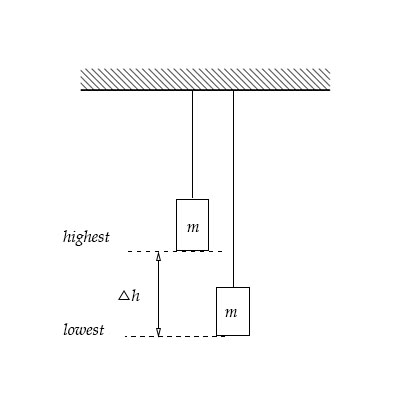# A spring, which has a spring constant k, is hung from the ceiling as shown below. A mass m = 3.00...

## Question:

A spring, which has a spring constant {eq}k {/eq}, is hung from the ceiling as shown below. A mass {eq}m = 3.00 \ kg {/eq} is added to the end of the spring and is then slowly lowered until equilibrium is reached. At this point, the bottom of the mass has been lowered a distance of {eq}\Delta h = 52.0 \ cm. {/eq}

a. What is the magnitude of the force being exerted by the spring when the system reaches equilibrium?

b. What is the spring constant of this spring?

c. How much energy is stored in the spring when equilibrium is reached?## Spring:

The external force required to stretch spring to a certain distance from its equilibrium position is equal to the product of spring constant and displacement. The work needed to do while extending or compressing the spring, which is proportional to the square of the displacement.

Become a Study.com member to unlock this answer! Create your account

Given data

• The mass which is added to the end of the spring is: {eq}m = 3\;{\rm{kg}} {/eq} .
• The extension is: {eq}\Delta h = 52\;{\rm{cm}} = 52...Practice Applying Spring Constant Formulas

from

Chapter 17 / Lesson 11
3.4K

In this lesson, you'll have the chance to practice using the spring constant formula. The lesson includes four problems of medium difficulty involving a variety of real-life applications.